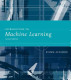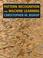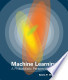### Machine Learning

#### Course Description

Machine Learning is a branch of artificial intelligence concerned with the design of algorithms that improve their performance based on empirical data. It has become one of the most active and exciting areas of computer science research, in large part because of its wide-spread applicability, ranging from data mining and pattern recognition to robotics, computer vision, computational biology, and computational linguistics. This course gives an in-depth coverage of the theory and principles of machine learning, and gives an overview of machine learning applications. The course covers two main approaches to machine learning: supervised learning (classification and regression) and unsupervised learning (clustering and dimensionality reduction).

#### General Competencies

Familiarity with supervised and unsupervised machine learning methods, classification and regression tasks. Understanding of generative, discriminative, parametric, and nonparametric models. Understanding of theoretical foundations of these models, the underlying assumptions, and their advantages and disadvantages. Capability to design and implement a machine learning system for classification, regression, or clustering and to evaluate its performance.

#### Learning Outcomes

1. define the basic concepts of machine learning
2. distinguish between generative and discriminative, parametric and nonparametric and probabilistic and nonprobabilistic models models
3. explain the theoretical assumptions, advantages, and disadvantages of basic machine learning algorithms
4. apply model selection and statistical evaluation of the learned model
5. apply various classification algorithms, inclusive generative, discriminative, and nonparametric ones
6. apply clustering algorithms and cluster validation
7. design and implement a machine learning method for classification/clustering and carry out its evalution
8. assess the suitability of a machine learning algorithm for a given task

#### Forms of Teaching

Lectures

Lectures are given for 13 weeks in two two-hour sessions per week.

Exams

Midterm exam and final exam.

Exercises

Recitations are given for 13 weeks in one-hour sessions as the need arises.

Consultations

Weekly office hours.

Programming Exercises

Programming assignments, demonstrated to the instructor or teaching assistant.

Other

Weekly homework assignments. Assignments are not reviewed and not graded; solutions are presented and discussed during recitation sessions.

Continuous Assessment Exam
Laboratory Exercises 30 % 30 % 0 % 30 %
Class participation 0 % 5 % 0 % 0 %
Mid Term Exam: Written 0 % 35 % 0 %
Final Exam: Written 0 % 35 %
Exam: Written 0 % 35 %
Exam: Oral 35 %
##### Comment:

Active participation in class is given 5% bonus points. Exams after the final exam are written and oral, together contributing 70% to the final grade. Students are required to score above 50% on written exam to be admitted to the oral exam.

#### Week by Week Schedule

1. Introduction to machine learning and motivation. Machine learning approaches. Machine learning tools. Supervised learning.
2. VC-dimension. Inductive bias. Generalization, overfitting and underfitting. Model selection.
3. Density estimation. Likelihood function. Estimators. Maximum likelihood estimator. MAP estimator.
4. Probabilistic generative models. Naive Bayes classifier. Seminaive Bayes classifer. Smoothing.
5. Regression. Least squares method. Generalized linear model of regression. Regularized regression.
6. Linear discriminative models. Geometry of a linear model. Perceptron. Multiclass schemes.
7. Logistic regression. Regularization. MaxEnt model.
8. Midterm exam.
9. Support vector machines. Kernel functions. SVM regresija.
10. Nonparametric methods. k-nearest neighbors algorithm. Decision trees. Nonparametric regression. Ansambli klasifikatora.
11. Classifier evaluation. Evaluation measures. Cross validation. Statistical testing.
12. Feature selection. Clustering. k-means algorithm.
13. Gaussian mixtures. Expectation maximization algorithm. Density-bsaed clustering. Hierarchical clustering. Cluster validation.
14. Dodatna tema / pozvano predavanje. Wrap-up.
15. Final exam.

#### Study Programmes

Computer Science (profile)
Theoretical Course (1. semester)
Information Processing (profile)
Recommended elective courses (3. semester)
Software Engineering and Information Systems (profile)
Theoretical Course (1. semester)

#### LiteratureEthem Alpaydin (2009.), Introduction to Machine Learning, The MIT PressChristopher M. Bishop (2007.), Pattern Recognition and Machine Learning, SpringerKevin P. Murphy (2012.), Machine Learning: A Probabilistic Perspective, The MIT Press
Jan Šnajder, Bojana Dalbelo Bašić (2012.), Strojno učenje -- skripta,
Trevor Hastie, Robert Tibshirani, Jerome Friedman (2009.), The Elements of Statistical Learning: Data Mining, Inference, and Prediction, Springer
(.), Info Theory, Inference, and Learning Algorithms;MacKay;2003;Cambridge University Press,

#### General

ID 103973
Winter semester
5 ECTS
L0 English Level
L1 e-Learning
45 Lectures
15 Exercises